# MD 4 1 MD 4 Message Digest 4

• Slides: 34MD 4 1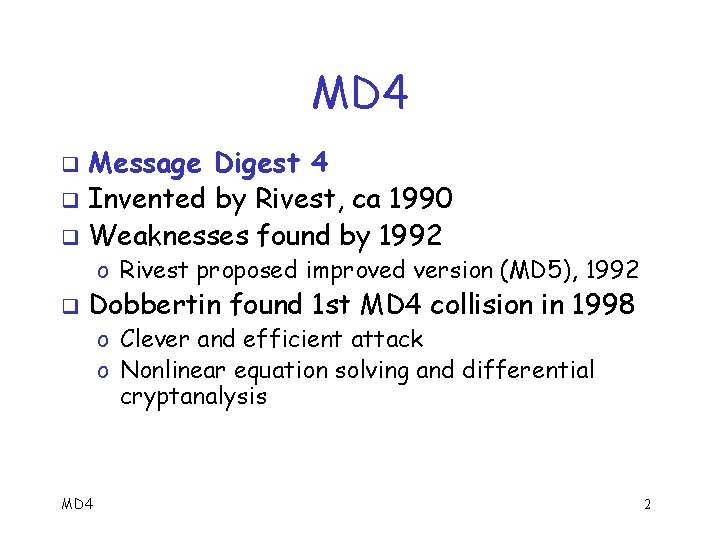MD 4 Message Digest 4 q Invented by Rivest, ca 1990 q Weaknesses found by 1992 q o Rivest proposed improved version (MD 5), 1992 q Dobbertin found 1 st MD 4 collision in 1998 o Clever and efficient attack o Nonlinear equation solving and differential cryptanalysis MD 4 2MD 4 Algorithm Assumes 32 -bit words q Little-endian convention q o Leftmost byte is low-order (relevant when generating “meaningful” collisions) Let M be message to hash q Pad M so length is 448 (mod 512) q o Single “ 1” bit followed by “ 0” bits o At least one bit of padding, at most 512 o Length before padding (64 bits) is appended MD 4 3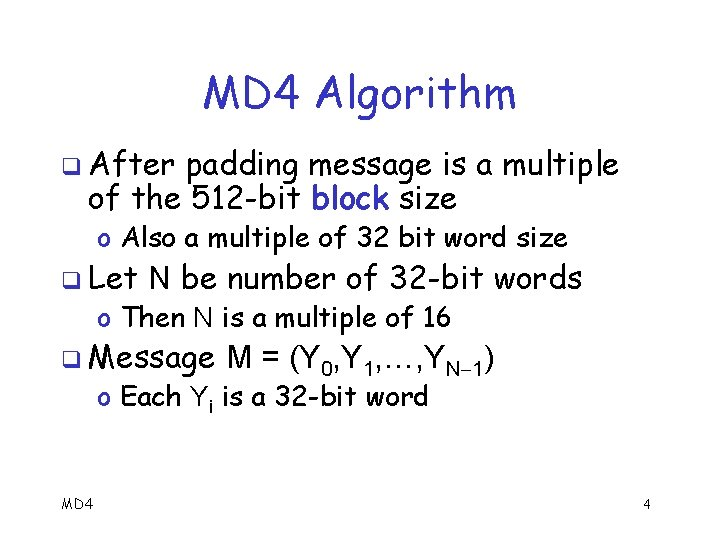MD 4 Algorithm q After padding message is a multiple of the 512 -bit block size o Also a multiple of 32 bit word size q Let N be number of 32 -bit words o Then N is a multiple of 16 q Message M = (Y 0, Y 1, …, YN 1) o Each Yi is a 32 -bit word MD 4 4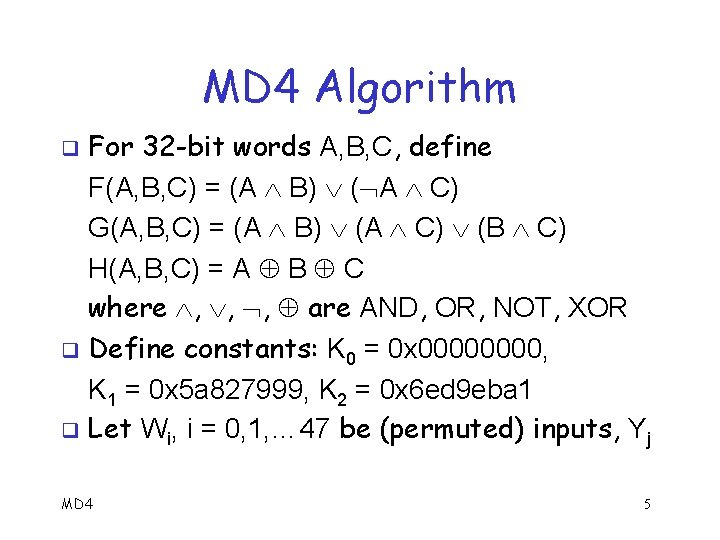MD 4 Algorithm For 32 -bit words A, B, C, define F(A, B, C) = (A B) ( A C) G(A, B, C) = (A B) (A C) (B C) H(A, B, C) = A B C where , , , are AND, OR, NOT, XOR q Define constants: K 0 = 0 x 0000, K 1 = 0 x 5 a 827999, K 2 = 0 x 6 ed 9 eba 1 q Let Wi, i = 0, 1, … 47 be (permuted) inputs, Yj q MD 4 5MD 4 Algorithm MD 4 6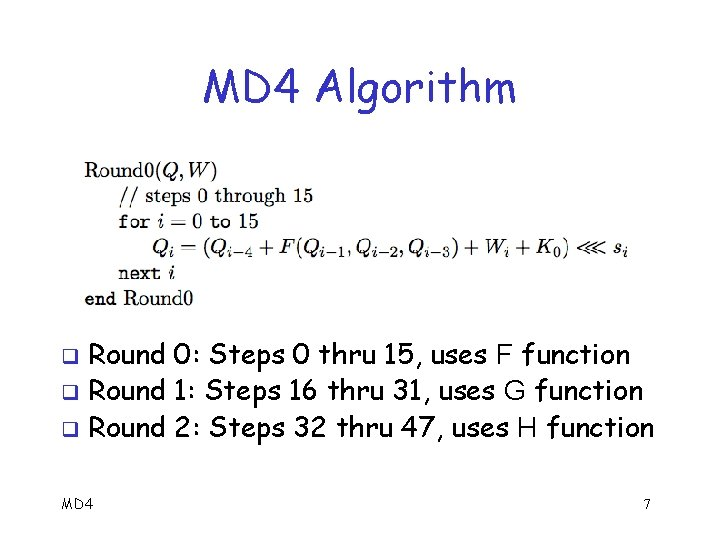MD 4 Algorithm Round 0: Steps 0 thru 15, uses F function q Round 1: Steps 16 thru 31, uses G function q Round 2: Steps 32 thru 47, uses H function q MD 4 7MD 4: One Step q Where MD 4 8Notation q Let MD 4 i…j(A, B, C, D, M) be steps i thru j o “Initial value” (A, B, C, D) at step i, message M q Note that MD 40… 47(IV, M) h(M) o Due to padding and final transformation q Let f(IV, M) = (Q 44, Q 47, Q 46, Q 45) + IV o Where “+” is addition mod 232, per 32 -bit word q Then f is the MD 4 compression function MD 4 9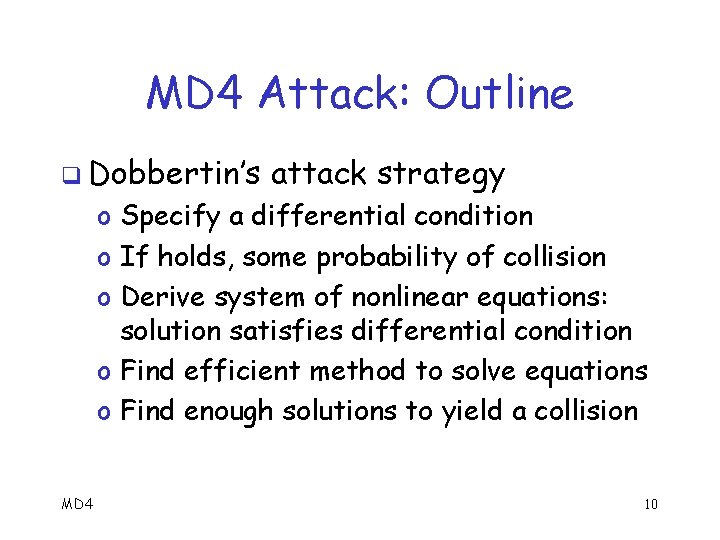MD 4 Attack: Outline q Dobbertin’s attack strategy o Specify a differential condition o If holds, some probability of collision o Derive system of nonlinear equations: solution satisfies differential condition o Find efficient method to solve equations o Find enough solutions to yield a collision MD 4 10MD 4 Attack: Motivation Find one-block collision, where M = (X 0, X 1, …, X 15), M = (X 0, X 1, …, X 15) q Difference is subtraction mod 232 q Blocks differ in only 1 word q o Difference in that word is exactly 1 q Limits avalanche effect to steps 12 thru 19 o Only 8 of the 48 steps are critical to attack! o System of equations applies to these 8 steps MD 4 11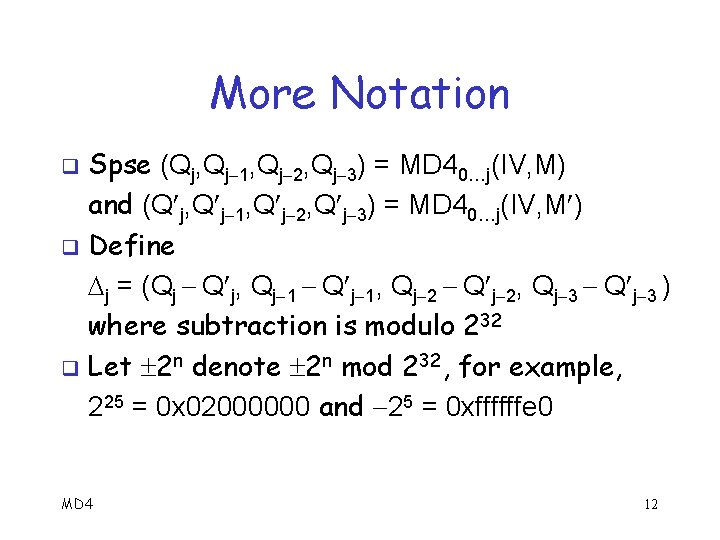More Notation Spse (Qj, Qj 1, Qj 2, Qj 3) = MD 40…j(IV, M) and (Q j, Q j 1, Q j 2, Q j 3) = MD 40…j(IV, M ) q Define j = (Qj Q j, Qj 1 Q j 1, Qj 2 Q j 2, Qj 3 Q j 3 ) where subtraction is modulo 232 q Let 2 n denote 2 n mod 232, for example, 225 = 0 x 02000000 and 25 = 0 xffffffe 0 q MD 4 12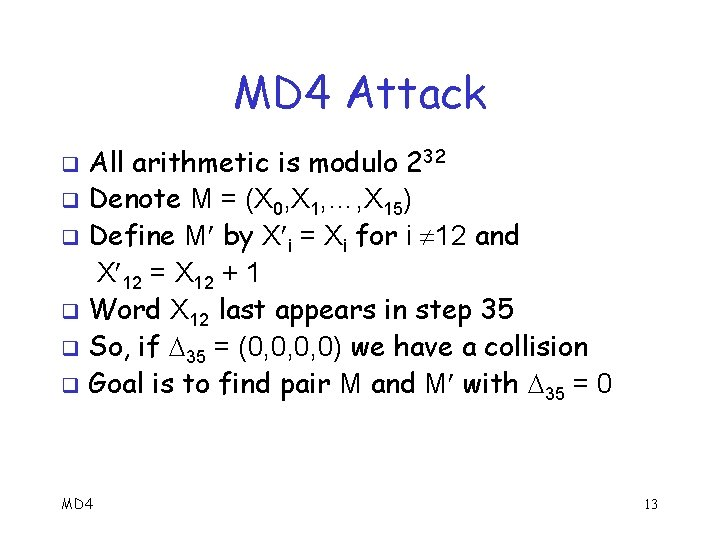MD 4 Attack All arithmetic is modulo 232 q Denote M = (X 0, X 1, …, X 15) q Define M by X i = Xi for i 12 and X 12 = X 12 + 1 q Word X 12 last appears in step 35 q So, if 35 = (0, 0, 0, 0) we have a collision q Goal is to find pair M and M with 35 = 0 q MD 4 13MD 4 Attack Analyze attack in three phases 1. Show: 19 = (225, 0, 0) implies probability at least 1/230 that the 35 condition holds q o Uses differential cryptanalysis o By solving system of nonlinear equations 2. “Backup” to step 12: We can start at step 12 and have 19 condition hold 3. “Backup” to step 0: Find collision MD 4 14MD 4 Attack q In each phase of attack, some words of M are determined q When completed, have M and M o Where M M but h(M) = h(M ) q Equation solving step is tricky part o Nonlinear system of equations o Must be able to solve efficiently MD 4 15Steps 19 to 35 q Differential phase of the attack q Suppose M and M as given above o Only differ in word 12 q Assume that 19 = (225, 0, 0) o And G(Q 19, Q 18, Q 17) = G(Q 19, Q 18, Q 17) q Then we compute probabilities of “ ” conditions at steps 19 thru 35 MD 4 16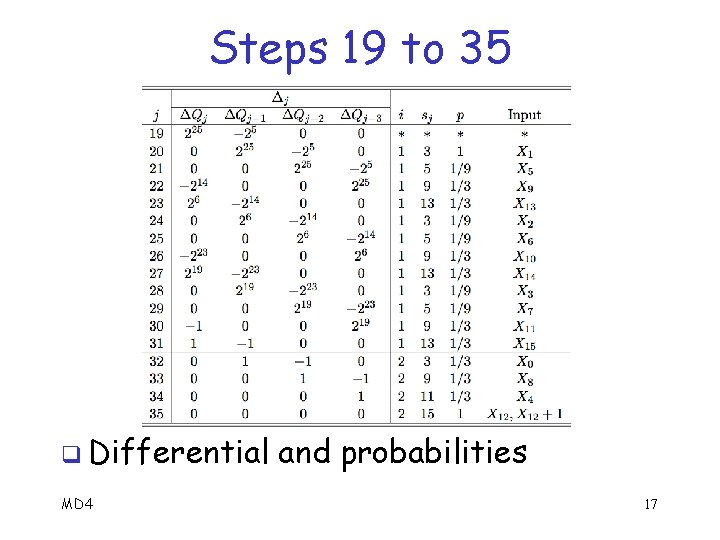Steps 19 to 35 q Differential MD 4 and probabilities 17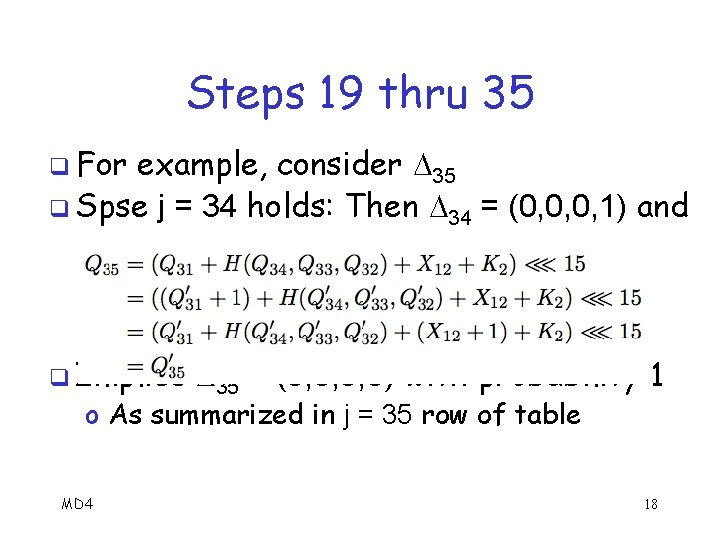Steps 19 thru 35 q For example, consider 35 q Spse j = 34 holds: Then 34 = (0, 0, 0, 1) and q Implies 35 = (0, 0, 0, 0) with probability 1 o As summarized in j = 35 row of table MD 4 18Steps 12 to 19 q Analyze steps 12 to 19, find conditions that ensure 19 = (225, 0, 0) o And G(Q 19, Q 18, Q 17) = G(Q 19, Q 18, Q 17), as required in differential phase q Step 12 to 19—equation solving phase q This is most complex part of attack o Last phase, steps 0 to 11, is easy MD 4 19Steps 12 to 19 q Info for steps 12 to 19 given here q If i = 0, function F, if i = 1, function G MD 4 20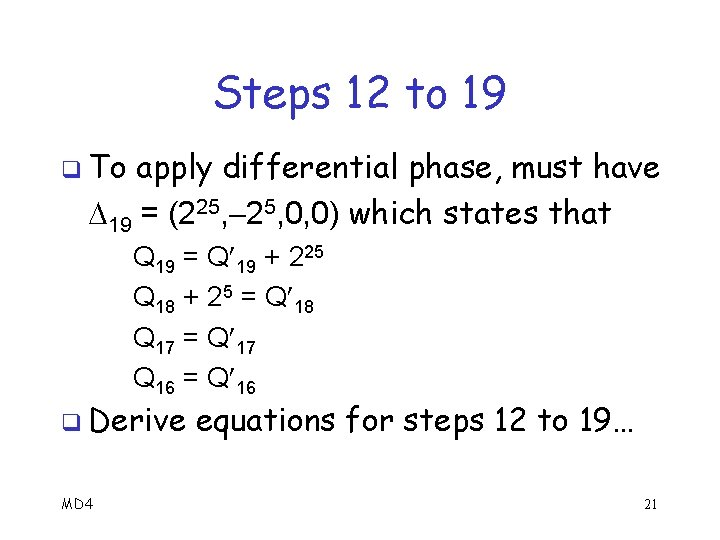Steps 12 to 19 q To apply differential phase, must have 19 = (225, 0, 0) which states that Q 19 = Q 19 + 225 Q 18 + 25 = Q 18 Q 17 = Q 17 Q 16 = Q 16 q Derive MD 4 equations for steps 12 to 19… 21Step 12 q At step 12 we have Q 12 = (Q 8 + F(Q 11, Q 10, Q 9) + X 12) <<< 3 Q 12 =(Q 8 + F(Q 11, Q 10, Q 9) + X 12) <<< 3 q Since X 12 = X 12 + 1 and (Q 8, Q 9, Q 10, Q 11) = (Q 8, Q 9, Q 10, Q 11) it follows that (Q 12 <<< 29) = 1 MD 4 22Steps 12 to 19 q Similar analysis for remaining steps yields system of equations: MD 4 23Steps 12 to 19 q To solve this system must find so that all equations hold q Given such a solution, we determine Xj for j = 13, 14, 15, 0, 4, 8, 12 so that we begin at step 12 and arrive at step 19 with 19 condition satisfied MD 4 24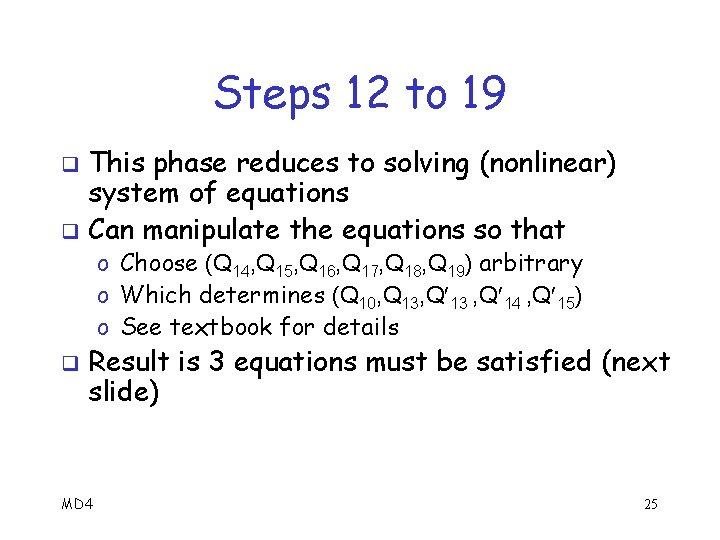Steps 12 to 19 This phase reduces to solving (nonlinear) system of equations q Can manipulate the equations so that q o Choose (Q 14, Q 15, Q 16, Q 17, Q 18, Q 19) arbitrary o Which determines (Q 10, Q 13 , Q 14 , Q 15) o See textbook for details q Result is 3 equations must be satisfied (next slide) MD 4 25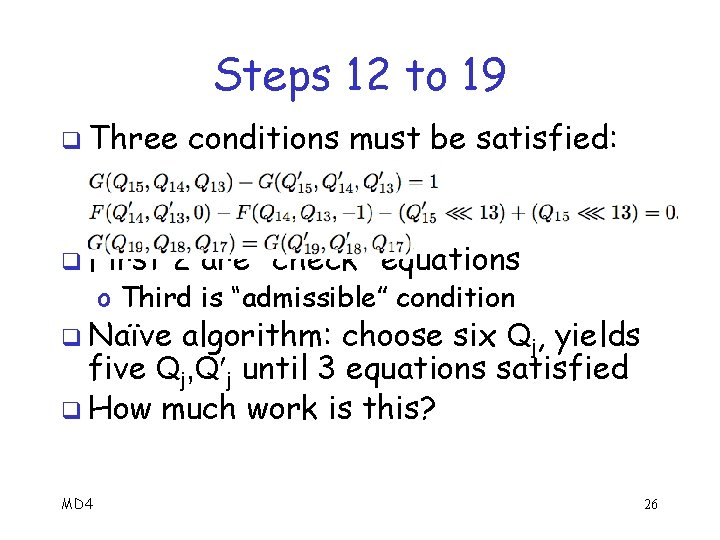Steps 12 to 19 q Three q First conditions must be satisfied: 2 are “check” equations o Third is “admissible” condition q Naïve algorithm: choose six Qj, yields five Qj, Q j until 3 equations satisfied q How much work is this? MD 4 26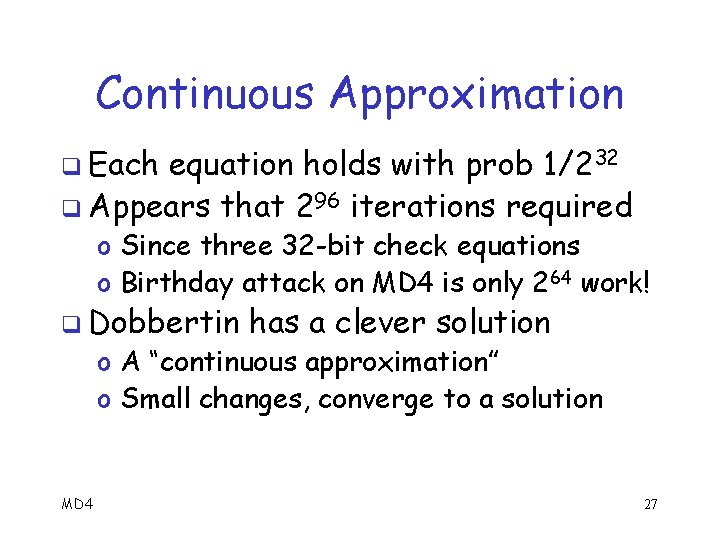Continuous Approximation q Each equation holds with prob 1/232 q Appears that 296 iterations required o Since three 32 -bit check equations o Birthday attack on MD 4 is only 264 work! q Dobbertin has a clever solution o A “continuous approximation” o Small changes, converge to a solution MD 4 27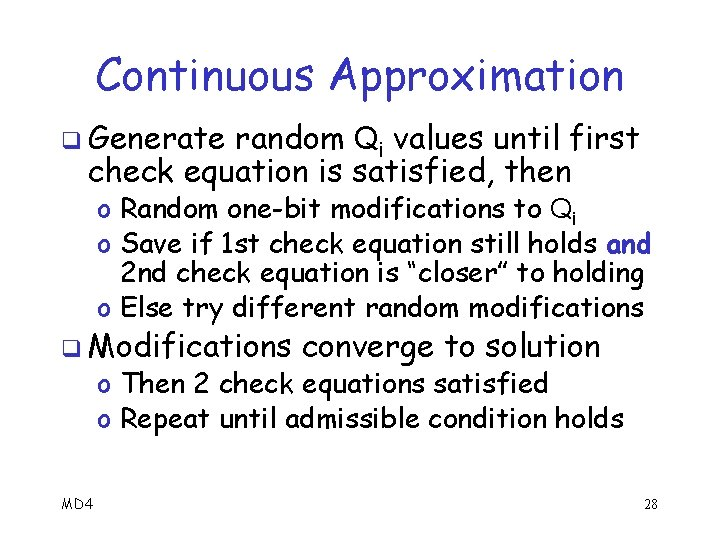Continuous Approximation q Generate random Qi values until first check equation is satisfied, then o Random one-bit modifications to Qi o Save if 1 st check equation still holds and 2 nd check equation is “closer” to holding o Else try different random modifications q Modifications converge to solution o Then 2 check equations satisfied o Repeat until admissible condition holds MD 4 28Continuous Approximation q For complete details, see textbook q Why does continuous approx work? o Small change to arguments of F (or G) yield small change in function value q What is the work factor? o Not easy to determine analytically o Easy to determine empirically (homework) o Efficient, and only once per collision MD 4 29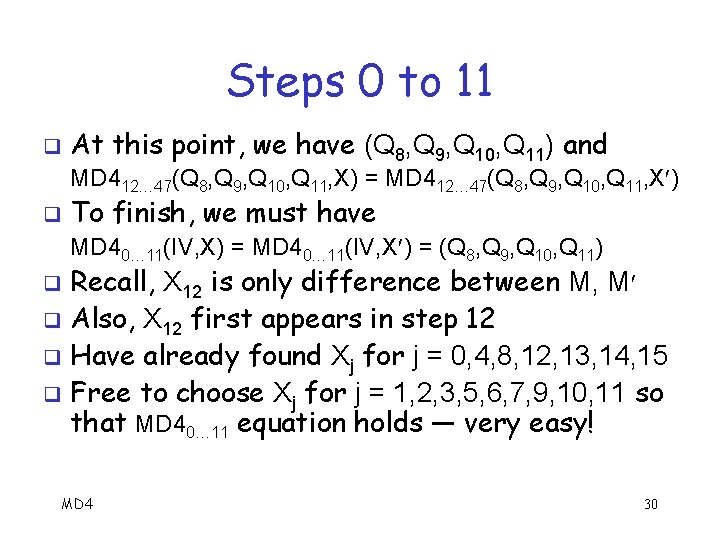Steps 0 to 11 q At this point, we have (Q 8, Q 9, Q 10, Q 11) and MD 412… 47(Q 8, Q 9, Q 10, Q 11, X) = MD 412… 47(Q 8, Q 9, Q 10, Q 11, X ) q To finish, we must have MD 40… 11(IV, X) = MD 40… 11(IV, X ) = (Q 8, Q 9, Q 10, Q 11) Recall, X 12 is only difference between M, M q Also, X 12 first appears in step 12 q Have already found Xj for j = 0, 4, 8, 12, 13, 14, 15 q Free to choose Xj for j = 1, 2, 3, 5, 6, 7, 9, 10, 11 so that MD 40… 11 equation holds — very easy! q MD 4 30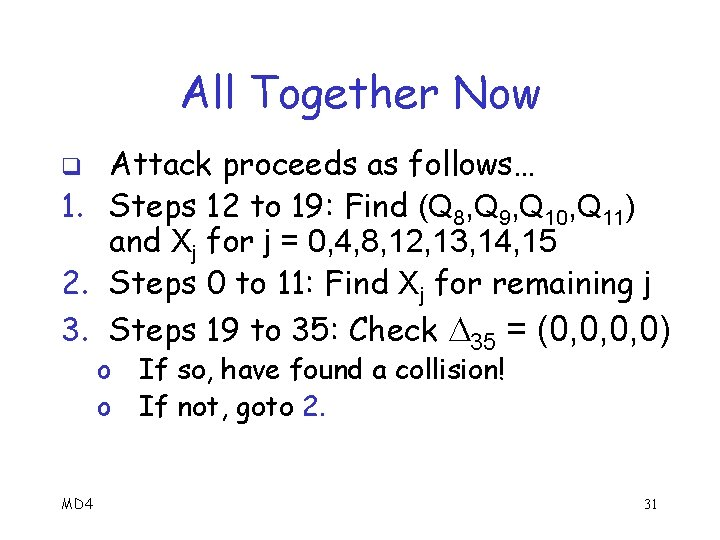All Together Now Attack proceeds as follows… 1. Steps 12 to 19: Find (Q 8, Q 9, Q 10, Q 11) and Xj for j = 0, 4, 8, 12, 13, 14, 15 2. Steps 0 to 11: Find Xj for remaining j 3. Steps 19 to 35: Check 35 = (0, 0, 0, 0) q o o MD 4 If so, have found a collision! If not, goto 2. 31Meaningful Collision q MD 4 collisions exist where M and M have meaning o Attack is so efficient, possible to find meaningful collisions q Let “ ” represent a “random” byte q Can find collisions on next slide… o Inserted for “security” purposes MD 4 32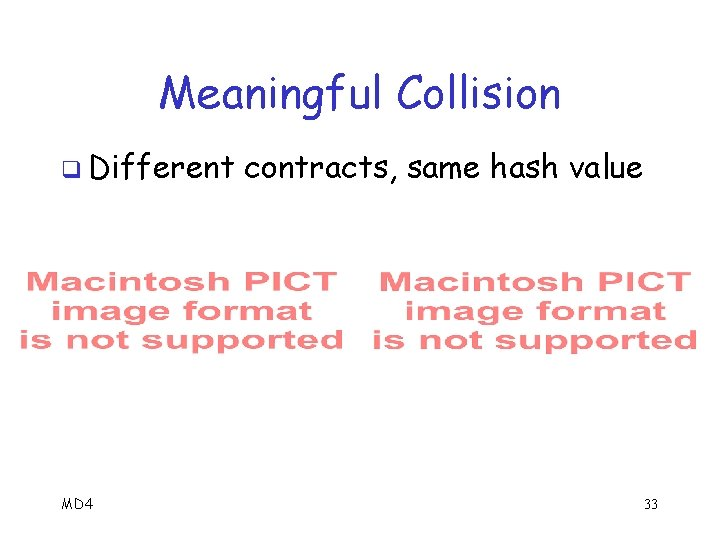Meaningful Collision q Different MD 4 contracts, same hash value 33MD 4 Conclusions q MD 4 weaknesses exposed early o Never widely used But took long time to find a collision q Dobbertin’s attack q o Clever equation solving phase o Only need to solve equations once/collision o Also includes differential phase q Next, MD 5… MD 4 34